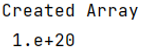# How to Format or Suppress Scientific Notation in NumPy?

Given a Pandas DataFrame, we have to format or suppress scientific notation. By Pranit Sharma Last updated : September 22, 2023

Scientific notations are nothing but the minimized long float values. Whenever we have a large number of values after the decimal point, the compiler converts it into the scientific notations.

## Format or Suppress Scientific Notation in NumPy

To convert the format of these notations or to suppress scientific notations in pandas DataFrame. We will use np.format_float_scientific() method. Consider the below-given syntax:

```np.format_float_scientific(
arr,
precision = 1,
exp_digits=2
)
```

By using the above syntax, we will be able to write a value in scientific notation. Here, arr is the array which we will create, note that the length of the arr will be 1.

Note

To work with NumPy, we need to import numpy package first, below is the syntax:

```import numpy as np
```

Let us understand with the help of an example,

## Python Program to Format or Suppress Scientific Notation in NumPy

```# Importing numpy package
import numpy as np

# Creating a Array
arr = np.array([10**20])

# Showing result in scientific notation
arr = np.format_float_scientific(arr, precision = 1, exp_digits=2)

# Display Created Array with scientific notations
print("Created Array\n",arr)
```

### Output

The output of the above program is: